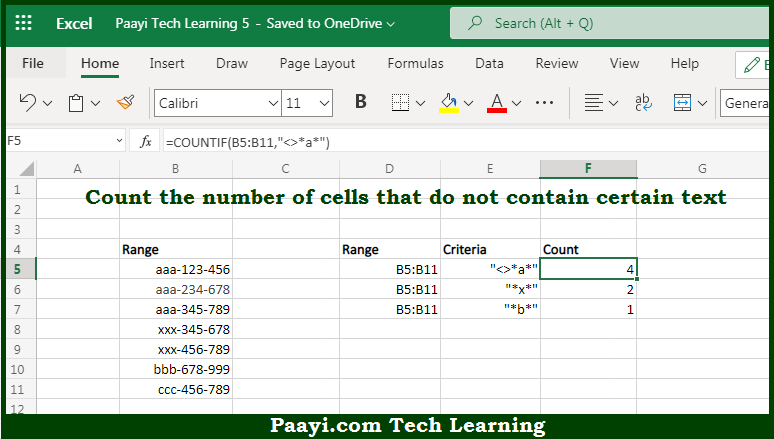# Learn How to Count Cells that Do Not Contain Specific Text in Microsoft Excel

Written by | 0 Comments | 563 Views

In this article, you will learn how to COUNT various things in Microsoft Excel using a single or combination of functions and its purpose in Microsoft Excel. You will also get to know how to count cells that do not contain specific text & generic formula

Count Cells that Do Not Contain Specific Text in Microsoft Excel

The main purpose of this formula is to count the number of cells that do not contain the specified text. Here we will learn how to count the range of cells not containing the specific text in Microsoft Excel.  That implies, with the help of a formula based on the  COUNTIF function with a wildcard you can able to count the number of cells in a range that does not contain a specific text. The generic form of the formula provided below "rng" is a range of cells, "txt" represents the text that cells should not contain, and "*" is a wildcard matching any number of characters. So, with the help of this formula, you can able to count the number of cells that do not contain the specified text.

General Formula to COUNT Cells that Do Not Contain Specific Text

=COUNTIF(rng,"<>*txt*")

The Explanation for the COUNT Cells that Do Not Contain Specific TextSo we know that with the help of the given formula above you can able to count the number of cells that do not contain the specified text. Here we will learn how to count the range of cells not containing the specific text in Microsoft Excel. As we know that, the  COUNTIF function is used to count the number of cells in the range that do not contain "a" by matching the content of each cell against the pattern "<>*a*", which is supplied as the criteria. The "*" symbol (the asterisk) is a wildcard in Excel that means "match any number of characters" and "<>" means "does not equal". That means this pattern will count any cell that does not contain "a" in any position. The count of cells that match this pattern will be returned as a number. So, with the help of this formula, you can able to count cells that do not contain specific text.